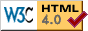[ Home | Tour | News | Photos | F.A.Q. | E.S.L. | Schools | Ham Radio | In the Press | Technical | Publications | Links | Papersat ]

# SUNSAT Technical Information

The following printouts give comms link budgets for various circumstances.
The Sunsat imager downlink was planned to give real time downlinking of areas close to Stellenbosch. At the University, we have a 4.5m diameter dish which we are modifying to include a LEO tracking capability. There are larger professional quality ground stations elsewhere in SA that can extend the communications slant range beyond the 1700km assumed for Stellenbosch.

The imager bit rate (and receiver noise bandwidth) is adjusted to maintain square pixels at the various orbit heights. Apogee and perigee cases are given below.

Sunsat's transmitter antenna is a 2-turn RHCP helix. Assume Tx gain = 0dBi to allow for cable and mispointing losses.

```For elavation = 30 degrees, Range = 2 * altitude

For 30 degrees elevation and 850km altitude, 33Mbit/s
Diam_metres = 4.5, GHz = 2.25, Eta = 0.6,    ===> Dish ant gain =38.29 dB_i
-------------------------------------------------------
Inputs
Carrier frequency                     GHz  = 2.25
Range                                  km  = 1700
Transmitter dBm                       dBm  = 37
Transmitter gain                      dBi  = 0
Receiving   gain                      dBi  = 38.29
Total noise temp, ant+cables+rx   Kelvins  = 100
Receiver IF bandwidth                  Hz  = 3.3e+007
-------------------------------------------------------
Microvolts into 50 Ohms                uV  = 8.115
Equivalent input noise power          dBm  = -103.4
IF Signal to noise ratio               dB  = 14.61
-------------------------------------------------------

For 30 degrees elevation and 520km altitude, 60Mbit/s
Diam_metres = 4.5, GHz = 2.25, Eta = 0.6,    ===> Dish ant gain =38.29 dB_i
-------------------------------------------------------
Inputs
Carrier frequency                     GHz  = 2.25
Range                                  km  = 1040
Transmitter dBm                       dBm  = 37
Transmitter gain                      dBi  = 0
Receiving   gain                      dBi  = 38.29
Total noise temp, ant+cables+rx   Kelvins  = 100
Receiver IF bandwidth                  Hz  = 6e+007
-------------------------------------------------------
Microvolts into 50 Ohms                uV  = 13.26
Equivalent input noise power          dBm  = -100.8
IF Signal to noise ratio               dB  = 16.28
-------------------------------------------------------

For 5 degrees elevation and 850km altitude, 3Mbit/s
Diam_metres = 4.5, GHz = 2.25, Eta = 0.6,    ===> Dish ant gain =38.29 dB_i
-------------------------------------------------------
Inputs
Carrier frequency                     GHz  = 2.25
Range                                  km  = 2400
Transmitter dBm                       dBm  = 37
Transmitter gain                      dBi  = 0
Receiving   gain                      dBi  = 38.29
Total noise temp, ant+cables+rx   Kelvins  = 100
Receiver IF bandwidth                  Hz  = 3e+006
-------------------------------------------------------
Microvolts into 50 Ohms                uV  = 5.748
Equivalent input noise power          dBm  = -113.8
IF Signal to noise ratio               dB  = 22.03
-------------------------------------------------------

-------------------------------------------------------
Inputs
Carrier frequency                     GHz  = 0.435
Range                                  km  = 2400
Transmitter dBm                       dBm  = 40
Transmitter gain                      dBi  = 0
Receiving   gain                      dBi  = 0
Total noise temp, ant+cables+rx   Kelvins  = 300
Receiver IF bandwidth                  Hz  = 1.5e+004
-------------------------------------------------------
Microvolts into 50 Ohms                uV  = 0.5113
Equivalent input noise power          dBm  = -132.1
IF Signal to noise ratio               dB  = 19.25
-------------------------------------------------------
-------------------------------------------------------
Inputs
Carrier frequency                     GHz  = 0.145
Range                                  km  = 2400
Transmitter dBm                       dBm  = 36
Transmitter gain                      dBi  = 0
Receiving   gain                      dBi  = 0
Total noise temp, ant+cables+rx   Kelvins  = 1000
Receiver IF bandwidth                  Hz  = 1.5e+004
-------------------------------------------------------